﻿ 宽带功率放大器记忆非线性失真补偿算法的仿真与性能优化 Simulation and Performance Optimization of Memory Nonlinear Distortion Compensation Algorithm for Wideband Power Amplifier

Hans Journal of Wireless Communications
Vol. 09  No. 02 ( 2019 ), Article ID: 29469 , 12 pages
10.12677/HJWC.2019.92007

Simulation and Performance Optimization of Memory Nonlinear Distortion Compensation Algorithm for Wideband Power Amplifier

Zhe Wang, Pu Miao*

Department of Communication Engineering, School of Electronic Information, Qingdao University, Qingdao Shandong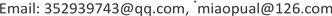Received: Mar. 6th, 2019; accepted: Mar. 20th, 2019; published: Mar. 27th, 2019ABSTRACT

The power amplifier (PA) is one of the most important nonlinear devices in wireless communication field. The memory-nonlinearity of the PA deteriorates the transmission performance of the communication system. The use of pre-distortion technology can reduce the system performance loss caused by the memory-nonlinearity of the PA. In this paper, the adaptive predistortion technique of PA’s memory-nonlinearity is studied and an adaptive predistortion model based on full kernel Volterra series is established. The parameters of the full-core Volterra predistortion model are identified by LS, RLS, LMS and Kalman filtering algorithms. The four algorithms are deeply optimized with the minimum mean square error (MSE) as an indicator. The simulation results show that the Kalman filtering algorithm has the best parameter identification accuracy. In the noise environment, the adaptive predistorter based on Kalman filter can still effectively compensate the memory-nonlinearity of PA. The research in this paper provides a reference for further understanding and research on adaptive predistortion technology of wireless communication systems.

Keywords:Power Amplifier, Predistortion Technology, Volterra Series, Parameter IdentificationCopyright © 2019 by author(s) and Hans Publishers Inc.

This work is licensed under the Creative Commons Attribution International License (CC BY).

http://creativecommons.org/licenses/by/4.0/1. 引言

PA作为无线通信系统的关键组成部分，其功能是将已调信号放大到所需功率并经天线发射。PA符合晶体管特性，在放大区内PA呈现线性，而随着输入信号功率的增加，PA将呈现非线性特性。同时随着带宽增加，记忆效应(即功放当前输出同时取决于当前的输入信号以及过去时刻的输入信号)也较为突出。PA的记忆非线性效应会导致通信信号的失真，带内误码率升高，带外频谱扩展，通信质量下降等问题  。为避免此类问题，常采用数字预失真技术对功率放大器的记忆非线性进行补偿，为保证对具有记忆效应的功率放大器非线性失真的补偿性能，数字预失真器的特性应与功率放大器的非线性特性及记忆特性完全相反，这些特性需通过建立功率放大器行为模型进行准确描述，功率放大器行为建模的本质是非线性系统辨识问题。

2. 功率放大器与预失真器技术

2.1. 功率放大器的非线性记忆特性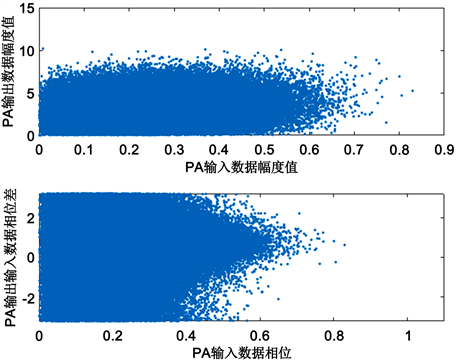Figure 1. AM-AM and AM-PM feature simulation

$\begin{array}{l}\phi \left(n\right)=\frac{{\alpha }_{\phi }{|x\left(n\right)|}^{2}}{1+{\beta }_{\phi }{|x\left(n\right)|}^{2}}+\mathrm{arg}\left[x\left(n\right)\right]\\ y\left(n\right)=\frac{{\alpha }_{A}|x\left(n\right)|}{1+{\beta }_{A}{|x\left(n\right)|}^{2}}+\mathrm{exp}\left[j\phi \left(n\right)\right]\end{array}$ (1)

$\begin{array}{l}{\alpha }_{A}=2.1587{\beta }_{A}=1.1517\\ {\alpha }_{\phi }=4.0330{\beta }_{\phi }=9.1040\end{array}$

2.2. 预失真技术原理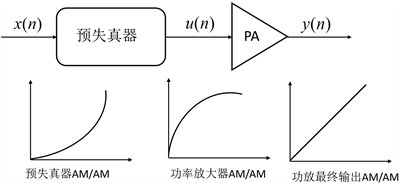Figure 2. Schematic diagram of predistortion technology

3. 预失真器的数学模型

3.1. Wiener模型

Wiener模型具有两箱式结构，由一个线性子系统和一个无记忆非线性系统级联而成，结构示意图如图3所示。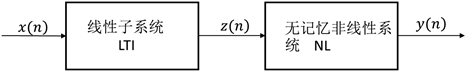Figure 3. Schematic diagram of the Wiener model structure

$\stackrel{˜}{y}\left(n\right)=\underset{k=1}{\overset{K}{\sum }}{\alpha }_{2k-1}\left[{|\underset{m=0}{\overset{M}{\sum }}h\left(m\right)\stackrel{˜}{x}\left(n-m\right)|}^{2\left(k-1\right)}\underset{m=0}{\overset{M}{\sum }}h\left(m\right)\stackrel{˜}{x}\left(n-m\right)\right]$ (2)

3.2. Hammerstein模型

Hammerstein模型与Wiener模型的结构相对称，可视作Wiener模型的逆模型，由一个无记忆非线性(NL)子系统与一个线性时不变级联而成，结构示意图如图4所示。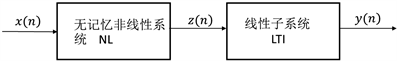Figure 4. Schematic diagram of the Hammerstein model structure

$\stackrel{˜}{y}\left(n\right)=\underset{m=0}{\overset{M}{\sum }}h\left(m\right)\underset{k=1}{\overset{K}{\sum }}{\alpha }_{2k-1}{|\stackrel{˜}{x}\left(n-m\right)|}^{2\left(k-1\right)}\stackrel{˜}{x}\left(n-m\right)$ (3)

3.3. Volterra级数模型

Volterra级数是Taylor幂级数的扩展，相当于在Taylor级数的基础上增加记忆项，它能较为全面地描述一个非线性系统并描述其记忆特性，非常适合动态非线性系统的建模，在记忆非线性功率放大器及数字预失真建模中得到非常广泛的应用，其时域表达式如式(4)：

$\begin{array}{l}y\left(t\right)={h}_{0}+\underset{n=1}{\overset{\infty }{\sum }}{z}_{n}\left[x\left(t\right)\right]\\ {y}_{n}\left[x\left(n\right)\right]=\underset{-\infty }{\overset{\infty }{\int }}\cdots \underset{-\infty }{\overset{\infty }{\int }}{h}_{n}\left({\tau }_{1},{\tau }_{2},\cdots {\tau }_{n}\right)x\left(t-{\tau }_{1}\right)x\left(t-{\tau }_{2}\right)\cdots x\left(t-{\tau }_{n}\right)\text{d}{\tau }_{1}\text{d}{\tau }_{2}\cdots \text{d}{\tau }_{n}\end{array}$ (4)

$\begin{array}{l}y\left(n\right)=\underset{{m}_{1}=0}{\overset{M}{\sum }}{h}_{1}\left({m}_{1}\right)x\left(n-{m}_{1}\right)+\underset{{m}_{1}=0}{\overset{M}{\sum }}\underset{{m}_{2}=0}{\overset{M}{\sum }}{h}_{2}\left({m}_{1},{m}_{2}\right)x\left(n-{m}_{1}\right)x\left(n-{m}_{2}\right)\\ \text{ }\text{ }\text{ }\text{ }\text{ }\text{ }\text{ }\text{ }\text{ }\text{ }\text{ }\text{ }\text{ }\text{ }\text{ }\text{ }\text{ }\text{ }\text{ }\text{ }\text{ }\text{ }\text{ }\text{ }\text{ }\text{ }\text{ }\text{ }\text{ }+\cdots +\underset{{m}_{1}=0}{\overset{M}{\sum }}\underset{{m}_{2}=0}{\overset{M}{\sum }}\cdots \underset{{m}_{p}=0}{\overset{M}{\sum }}{h}_{k}\left({m}_{1},{m}_{2},\cdots ,{m}_{k}\right)x\left(n-{m}_{1}\right)x\left(n-{m}_{2}\right)\cdots x\left(n-{m}_{k}\right)\end{array}$ (5)

4. Volterra预失真器模型辨识

4.1. 预失真器参数辨识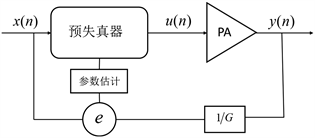Figure 5. Predistorter direct learning structure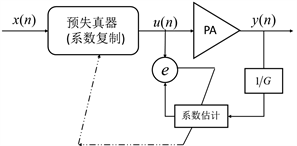Figure 6. Predistorter indirect learning structure

4.2. 最小二乘算法(LS算法)

$J\left({a}_{0},{a}_{1},\cdots {a}_{n}\right)=\underset{k=1}{\overset{N}{\sum }}{e}^{2}\left(k\right)=\underset{k=1}{\overset{N}{\sum }}{\left[y\left(k\right)-\stackrel{^}{y}\left(k\right)\right]}^{2}$ (6)

LS算法参数估计值 ${a}_{0},{a}_{1},\cdots {a}_{n}$ 满足：

${J}_{LS}=\mathrm{min}\left[J\left({a}_{0},{a}_{1},\cdots {a}_{n}\right)\right]$ (7)

$\begin{array}{l}{X}_{k}={\left(1,{x}_{1}\left(k\right),\cdots {x}_{n}\left(k\right)\right)}^{T}\\ \theta ={\left({a}_{0},{a}_{1},\cdots ,{a}_{n}\right)}^{T}\end{array}$ (8)

$y\left(k\right)={X}_{k}{}^{T}\theta +e\left(k\right)$ (9)

y(k)的向量表达式也可用矩阵表示：

${y}_{N}={X}_{N}\theta +{e}_{N}$ (10)

$J\left(\theta \right)={\left({y}_{N}-{X}_{N}\theta \right)}^{T}\left({y}_{N}-{X}_{N}\theta \right)$ (11)

${X}_{N}^{T}{X}_{N}$ 为满秩矩阵，可得最小二乘解为：

$\theta ={\left({X}_{N}^{T}{X}_{N}\right)}^{-1}{X}_{N}^{T}{y}_{N}$ (12)

4.3. 递归最小二乘法(RLS算法)

LS算法是通过计算矩阵 ${\left({X}_{N}^{T}{X}_{N}\right)}^{-1}$ 一次处理所得。递归算法指在每取得一新数据时，就根据此数据对原估计量进行修正得到新的估计量。引入一个加权因子 $\lambda$ 对式(6)进行修正：

$\epsilon \left(n\right)=\underset{k=1}{\overset{n}{\sum }}{\lambda }^{n-k}{e}^{2}\left(k\right)$ (13)

RLS算法是通过 $n-1$ 时刻数据去估计n时刻数据。最常用的递归最小二乘算法为：

$\begin{array}{l}{\stackrel{^}{\theta }}_{N+1}={\stackrel{^}{\theta }}_{N}+\frac{{P}_{N}{x}_{N+1}}{{x}_{N+1}^{T}{P}_{N}{x}_{N+1}+1}\left[y\left(n+1\right)-{x}_{N+1}^{T}{\stackrel{^}{\theta }}_{N}\right]\\ {P}_{N+1}={P}_{N}-\frac{{P}_{N}{x}_{N+1}{x}_{N+1}^{T}{P}_{N}}{{x}_{N+1}^{T}{P}_{N}{x}_{N+1}+1}\end{array}$ (14)

4.4. 最小均方算法(LMS算法)

RLS算法简化形式为： ${\stackrel{^}{\theta }}_{N+1}={\stackrel{^}{\theta }}_{N}+Kerror$ ，用 $\mu {X}_{N}$ 代替增益矩阵 $K$ 即为LMS算法：

${\stackrel{^}{\theta }}_{N+1}={\stackrel{^}{\theta }}_{N}+\mu {X}_{N}error$ (15)

$\begin{array}{l}\stackrel{^}{y}\left(n\right)={X}^{T}\left(n\right)\theta \left(n\right)\\ e\left(n\right)=y\left(n\right)-\stackrel{^}{y}\left(n\right)\\ \theta \left(n+1\right)=\theta \left(n\right)-2\mu X\left(n\right)e\left(n\right)\end{array}$ (16)

4.5. 卡尔曼滤波算法(Kalman滤波算法)

$n-1$ 时刻参数辨识值 ${\stackrel{^}{\theta }}_{N-1}$ 预测N时刻的参数辨识值 ${\stackrel{^}{\theta }}_{N}$ ，有：

${\stackrel{^}{\theta }}_{N}{}^{\text{'}}=A{\stackrel{^}{\theta }}_{N-1}+B{\mu }_{N-1}$ (17)

${P}_{N}{}^{\text{'}}\text{=}A{P}_{N-1}{A}^{T}+Q$ (18)

$K=\frac{{P}_{N}{}^{\text{'}}{X}_{N}^{T}}{{X}_{N}{P}_{N}{}^{\text{'}}{X}_{N}^{T}+\lambda }$ (19)

${\stackrel{^}{\theta }}_{N}={\stackrel{^}{\theta }}_{N}{}^{\text{'}}+K\left({y}_{N}-{X}_{N}{\stackrel{^}{\theta }}_{N}{}^{\text{'}}\right)$ (20)

${P}_{N}={P}_{N}{}^{\text{'}}+K{X}_{N}{P}_{N}+Q$ (21)

5. 仿真分析

$\begin{array}{l}MSE=\frac{1}{n}\underset{k=1}{\overset{K}{\sum }}{\left({y}_{k}-{\stackrel{^}{y}}_{k}\right)}^{2}\\ NMSE=10\mathrm{log}MSE\left(\text{dB}\right)\end{array}$ (22)

MSE越小表示模型精确有更好的精确度。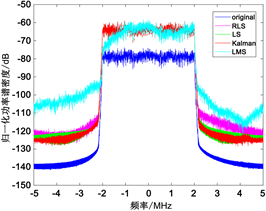Figure 7. Comparison of power spectral densities obtained by different parameter identification algorithmsTable 1. Model accuracy for different algorithm identification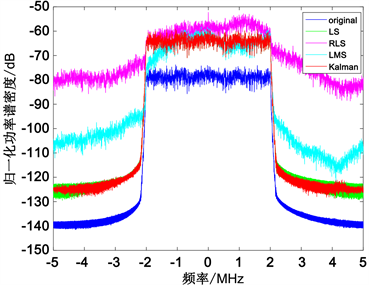Figure 8. Power spectral density of a signal passing through DPD and PA at 30 dB noiseTable 2. Model accuracy for different algorithm identification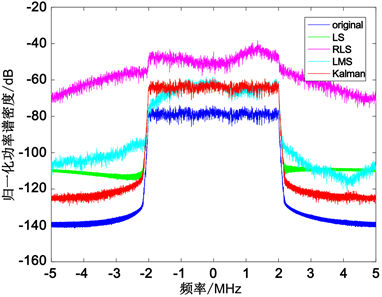Figure 9. Model output power spectral density at 20 dB noiseTable 3. MSE of model identification at 20 dB noise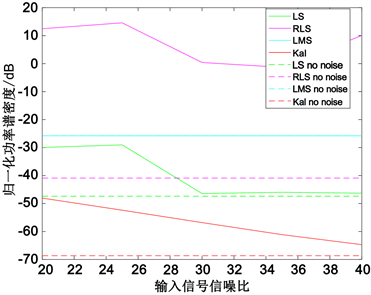Figure 10. MSE of the model under different noise conditions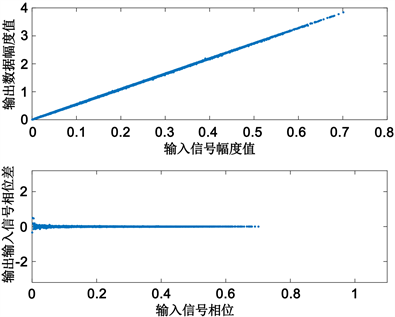Figure 11. Amplification characteristics of DPD-PA identified by Kalman filtering algorithm

6. 结论

Simulation and Performance Optimization of Memory Nonlinear Distortion Compensation Algorithm for Wideband Power Amplifier[J]. 无线通信, 2019, 09(02): 47-58. https://doi.org/10.12677/HJWC.2019.92007

1. 1. Kenney, J.S. and Fedorenko, P. (2006) Identification of RF Power Amplifier Memory Effect Origins Using Third-Order Intermodu-lation Distortion Amplitude and Phase Asymmetry. International Microwave Symposium Digest.

2. 2. Billings, S.A. (1980) Book Review: The Volterra and Wiener Theories of Nonlinear Systems. IEE Proceedings D Control Theory and Applications, 127, 236.
https://doi.org/10.1049/ip-d.1980.0039

3. 3. Zhu, A., Draxler, P.J., Yan, J.J., et al. (2008) Open-Loop Digital Predistorter for RF Power Amplifiers Using Dynamic Deviation Reduction-Based Volterra Series. IEEE Transactions on Microwave Theory and Techniques, 56, 1524-1534.
https://doi.org/10.1109/TMTT.2008.925211

4. 4. Zhou, D. and Debrunner, V.E. (2012) Novel Adaptive Nonlinear Predistorters Based on the Direct Learning Algorithm. Microprocessors, 55, 120-133.

5. 5. Kwong, R.H. and Johnston, E.W. (1992) A Variable Step Size LMS Algorithm. IEEE Transactions on Signal Processing, 40, 1633-1642.
https://doi.org/10.1109/78.143435

6. 6. 张玉梅, 南敬昌. 基于Saleh函数的功放行为模型研究[J]. 微电子学与计算机, 2010, 27(12): 121-123.

7. 7. 金哲. 宽带通信中有记忆射频功率放大器的建模与预失真方法[D]: [博士学位论文]. 杭州: 浙江大学, 2007.

8. 8. Morgan, D.R., Ma, Z., Kim, J., et al. (2006) A Generalized Memory Polynomial Model for Digital Predistortion of RF Power Amplifiers. IEEE Transactions on Signal Processing, 54, 3852-3860.
https://doi.org/10.1109/TSP.2006.879264

9. 9. 王栋. 适用于宽带功率放大器的自适应数字预失真技术研究[D]: [硕士学位论文]. 南京: 南京理工大学, 2017.

10. 10. 李宁. LMS自适应滤波算法的收敛性能研究与应用[D]: [博士学位论文]. 哈尔滨: 哈尔滨工程大学, 2009.

11. 11. 高明明, 湛素丽, 南敬昌. 稀疏的归一化功放模型及预失真应用[J]. 计算机应用研究, 2017, 34(10): 3032-3035.

12. NOTES

*通讯作者。